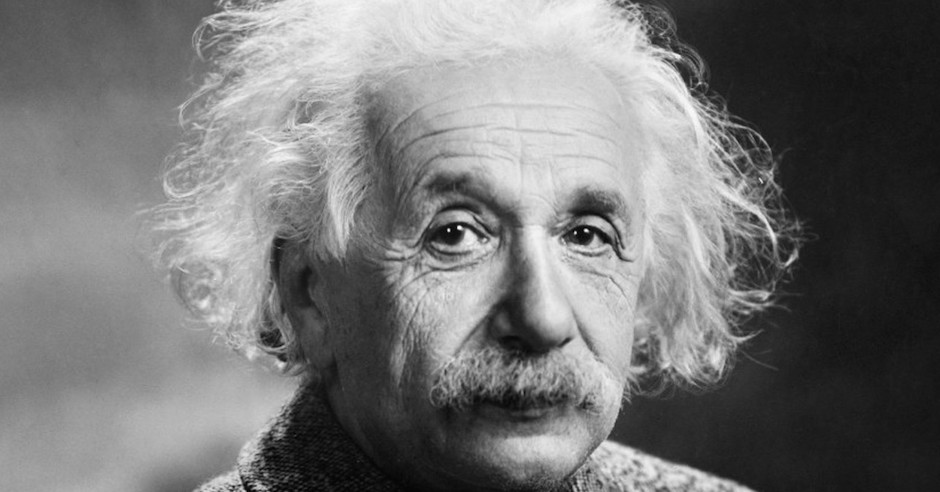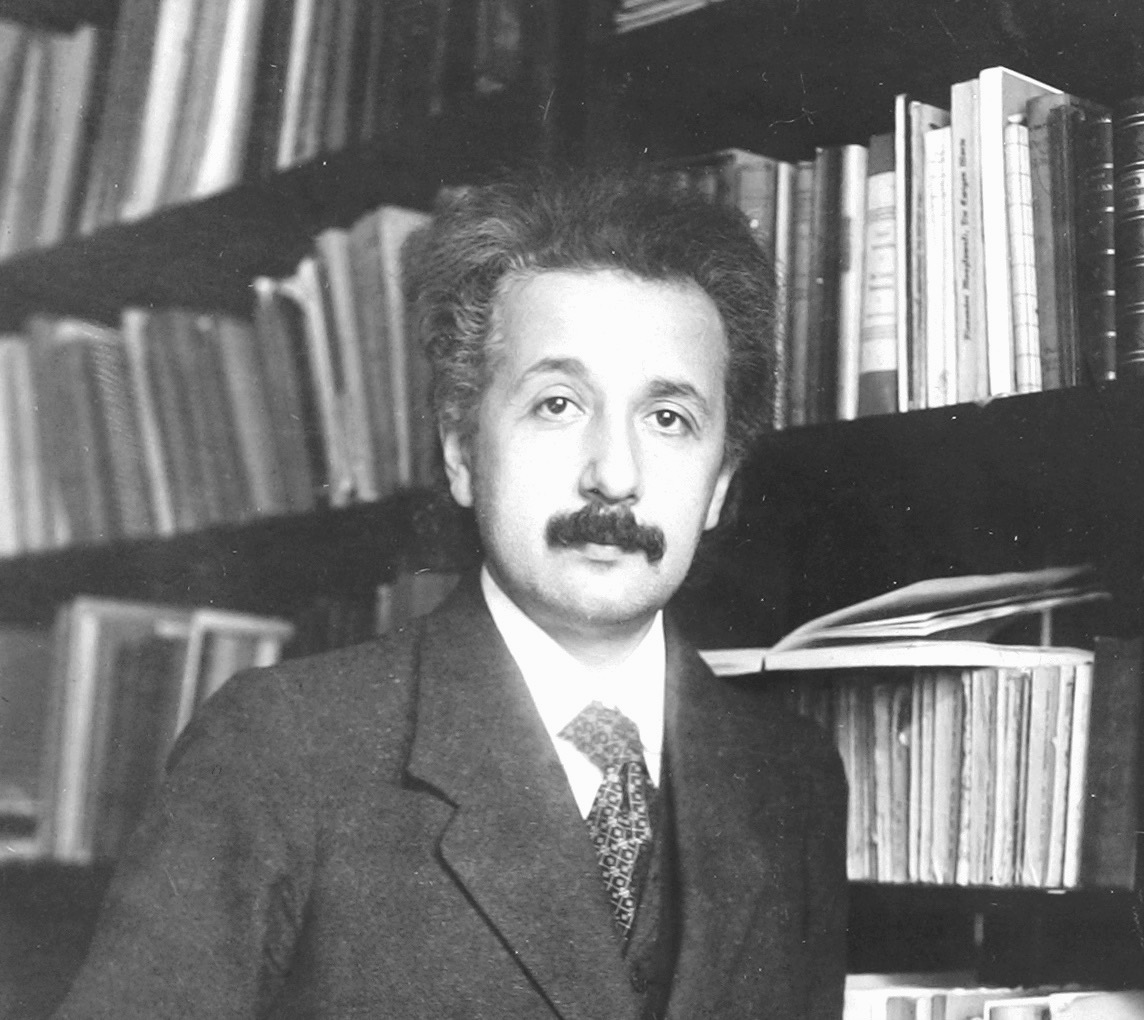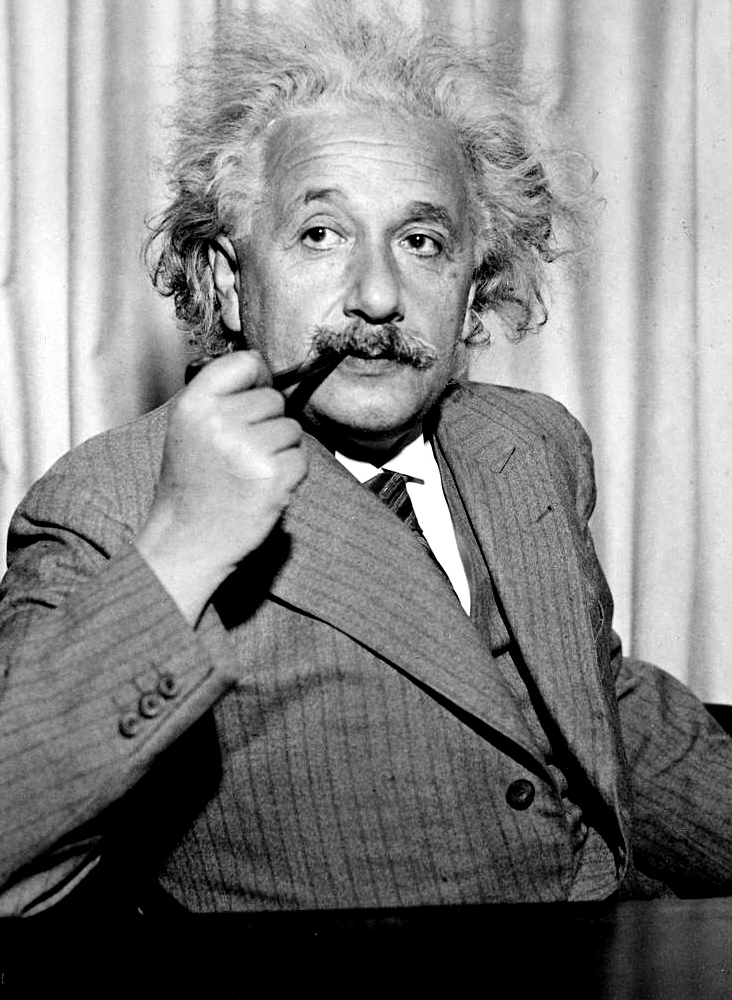# The Enduring Genius of Albert EinsteinBy Rick Larson Jr.

Albert Einstein (March 14th, 1879- April 18th, 1955) is one of the most talked about scientists, with some of the most highly regarded findings, and for good reason. Born March 14, 1879, the German born theoretical physicist is best known for his theory of Special Relativity, which created one of two current major pillars of modern physics and the famous equation E=mc2, also known as the mass-energy equivalence.

E=MC2

Most people have heard of E = mc², but few understand the importance of what it means. In 1921 Einstein received the Nobel Peace Prize for “his services to theoretical physics, and especially for his discovery of the law of the photoelectric effect”, which was a huge leap in the evolution of the quantum theory.

Without this revolutionary finding, building the atom bomb would have not been possible, along with many other things that are concerned with the fundamental concepts of physics that we know as true today.

In a basic sense, the equation shows the relationship of an object’s mass, to the amount of energy that is within that object. (E) is energy, which equals (m), the mass of an object multiplied by (c), a constant which stands for the speed of light (186,000 miles per second).

To put this enormous number in perspective, the next closest star to Earth is Alpha Centauri, at a relatively close distance of about 4.4 light years away, or 2.587×10^13 miles away.

In terms of the universe, this is a tiny number, but on earth this is monumental. That is 25.87 TRILLION miles away! This equation was and continues to be, revolutionary because it allows us to find the total energy of an object, by knowing just its mass since the speed of light (in a vacuum) is constant.

If you were to be able to travel at speeds close to the speed of light, objects in front of you would look smaller, or shrunken with a blue tone, while objects behind you would be of a red tint and seem enlarged.

This is called the Doppler effect and was first described by Christian Andreas Doppler, which led to the finding of the redshift, discovered by Edwin Hubble.

He was the first to describe the redshift phenomenon and correlate to our ever-expanding universe. Redshift of an object is defined as the change in the wavelength of the light divided by the wavelength that the light would have if the source was not moving, called the rest wavelength.

When an object moves away, the light wavelength is lengthened and moves towards the red end of the spectrum. When an object moves closer, the wavelengths shorten and move towards the blue end of the spectrum.Relativity

The Theory of Special Relativity was just as groundbreaking as the mass-energy equivalence. In the year 1905, Einstein was able to determine that the laws of physics are universal for any, and all non-accelerating observers, with the speed of light within a vacuum (space) being independent of its observers, and their motion.

Over the next decade, Einstein would try to include acceleration in this theory, and in 1915 he was able to. The science genius was able to show that objects with a very large mass could cause a distortion in the relationship between space and time, which is then felt as gravity. This is an incredible find, especially for it being the beginning of the 20th Century!